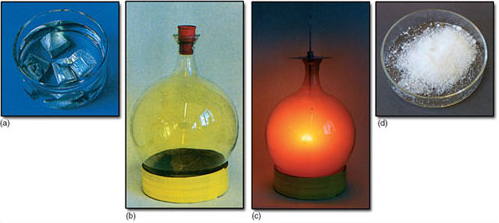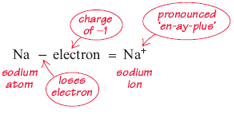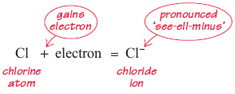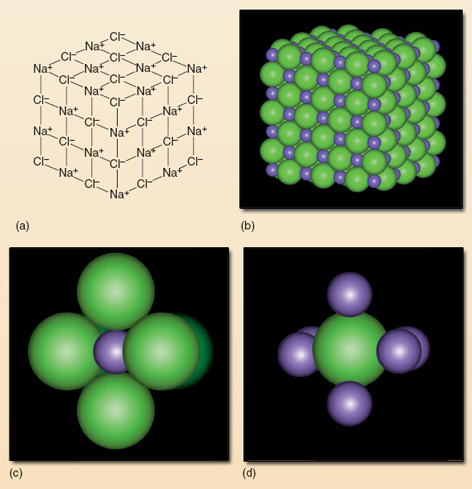Water for life

This free course is available to start right now. Review the full course description and key learning outcomes and create an account and enrol if you want a free statement of participation.

Free course

# 7 Ions and ionic bonding

This section returns to bonding - the way in which atoms are joined to each other. You have already met one type of bonding involving covalent bonds, which is found in molecules. However, this is not the only bonding found in compounds. In this section you will look at ionic bonding and the ionic compounds that contain such bonding. What is the main difference between the covalent compounds you met in Section 5 and ionic compounds? Are there any similarities between these two enormous families of chemical compounds?

Glucose is a covalent compound and sodium chloride is an ionic compound. You meet glucose in solution in everyday life as it is the sugar in many sweet drinks (and is closely related to ordinary table sugar). You are certainly familiar with sodium chloride as it is the table salt used in kitchens. The formulas of both compounds tell you which atoms have combined together to make them. You can work this out for yourself in the following question.

## Question 27

The formula of glucose is C6H12O6 and the formula of salt is NaCl. Which elements are combined to make glucose? Which elements are combined to make salt? Use Table 5 to help you if necessary.

The covalent compound glucose is formed from the elements carbon, hydrogen and oxygen. The ionic compound sodium chloride is formed from the elements sodium and chlorine.

If you bought some glucose tablets from a pharmacy or a sports shop and crushed them, you would have a fine, white, sweet powder - certainly not like the elements that form it: black carbon, gaseous hydrogen and gaseous oxygen.

Equally, sodium chloride is vastly different from the elements that combine to make it (Figure 23). Sodium chloride is a white solid that is used in food. Yet chlorine is an enormously reactive green gas, deadly poisonous in concentrated form, and an excellent disinfectant when dissolved in water, such as in swimming pools. You probably haven't seen sodium as this silvery metal catches fire in air and almost explodes in water. Once again, the compound - an ionic compound in this case - is completely different from its constituent elements.Figure 23 (a) Sodium metal (immersed in oil to prevent reaction with oxygen and moisture in the air); (b) chlorine gas; (c) the reaction between sodium and chlorine; (d) sodium chloride (common table salt)

If covalent and ionic compounds are similar in that they are unlike the elements that form them, in what key way do they differ? The answer is in the nature of the bonding. To understand ionic bonding, you need to recall what you learned in Section 4 about the internal structure of atoms.

## Question 28

Which kind of charge does an electron carry? In which way is the overall electrical neutrality of atoms achieved?

Each electron carries one negative charge that is represented as −1. An atom is neutral because the number of protons (each of which bears a positive or +1 charge) exactly equals the number of electrons.

Now apply this to the elements that are in common salt. Table 3 lists the number of electrons (and hence protons) in five elements. Table 6 shows the comparable information about sodium and chlorine.

## Table 6 The number of electrons and protons in sodium and chlorine

Element No. of electrons No. of protons
sodium 11 11
chlorine 17 17

How do these two elements bond together? Picture a sphere representing a sodium atom. The sphere isn't solid: it has a tiny central nucleus (with a charge of +11) and a cloud of electrons arranged in layers (with a total charge of −11). In many chemical reactions, an atom of sodium very easily loses one electron from the outer layer of this cloud of electrons. The loss of a single electron from a sodium atom makes it into a particle bearing a net positive charge of +1. This positive particle is termed an ion (pronounced 'eye-on'). By taking away one electron from a sodium atom, a sodium ion is formed:This equation can be easily explained. The sodium atom is electrically neutral; it comprises 11 protons (+11) and 11 electrons (−11). When it loses one electron with a charge of −1, by arithmetic, the particle that is left must have a charge of +1. Note that this is represented by the symbol for sodium with a small superscript 'plus' sign.

Now picture a chlorine atom. This is electrically neutral: it has 17 electrons and 17 protons. In its reactions a chlorine atom likes to gain one electron. Following the same line of reasoning as before, adding one electron to this atom gives one extra negative charge. Thus a chlorine ion (usually called a chloride ion) is formed. Once again, the ion is represented by the symbol for the element followed by the charge written as a superscript. Thus:The next question covers the same ground but looks at the change from atom to ion in overall terms.

## Question 29

Look back at chlorine in Table 6. (a) What charge is in the nucleus after the electron is gained? (b) What total charge is there in the remaining set of electrons? (c) What, therefore, is the net charge on the ion as a whole?

(a) The protons are undisturbed; so the charge in the nucleus is still +17. (b) The electrons are one more than before; so their charge is −18. (c) The outcome of +17 and −18 is −1. So the net charge on the ion is −1.

The fact that a sodium atom likes to lose an electron and a chlorine atom likes to gain an electron means that they have a great potential for satisfying each other's 'needs'. This is what lies behind the violence of the reaction between shiny sodium metal and green poisonous chlorine gas (Figure 23(c)). If you dropped a lump of sodium into a container of chlorine gas (behind armoured glass as the reaction is very violent and involves flames) electron transfer occurs. Each sodium atom loses an electron (so forming Na+) and each chlorine atom gains one (so forming Cl). On the bottom of the container of chlorine gas, there would be a trace of white powder: the compound sodium chloride, common table salt. The Na+ ions and the Cl ions formed in the reaction attract each other in a similar way to a comb attracting paper. The positive ions are strongly attracted to the negative ions.

## Question 30

Suppose one billion sodium atoms lost one electron each to one billion chlorine atoms. (a) How many electrons move from Na atoms to Cl atoms? (b) How many sodium ions (Na+) are formed? (c) How many chloride ions (Cl) are formed? (d) What is the net charge on the resulting sodium chloride? (e) How many molecules are formed?

(a) one billion; (b) one billion; (c) one billion; (d) zero. The answer to (e) may surprise you: none!

The net charge on any ionic compound is always zero. The atoms, after all, start out neutral. All that happens is that some electrons move from one place to another. The total charge on the positive ions exactly equals the total charge on the negative ions.

The answer to (e) raises an important point. In ionic compounds such as salt, it is the attraction between the negatively charged chloride ions and the positively charged sodium ions which holds the substance together. This attraction operates in all directions, unlike the bonding in covalent compounds where the linking is directly between the atoms. Figure 24 shows this in a magnified piece of salt.Figure 24 A magnified piece of salt. (a) Here the solid lines represent the electrical attraction between every Na+ and each of its neighbouring Cl ions. In fact, this is a two-dimensional representation of a more complex three-dimensional structure. (b) The ions are shown in their space-filling representation. The larger spheres are chloride ions and the smaller ones sodium ions. (c) and (d) illustrate the 'ion packing' structure within sodium chloride - that each sodium ion is surrounded by 6 chloride ions, and that each chloride ion is surrounded by 6 sodium ions, respectively

A distinct molecule of sodium chloride cannot exist because each sodium ion is attracted to more than one chloride ion and vice versa. Thus drawing directional bonds between atoms, as you did for the covalent molecules in Section 5, would be meaningless.

So far, you have considered the Na+ ion and the Cl ion which have lost and gained one electron, respectively. Some atoms like to lose more than one electron or gain more than one electron. For example, atoms of the element calcium (another very reactive metal) always lose two electrons, so the calcium ion is always Ca++ normally written as Ca2+ (pronounced 'see-ay-two-plus'). In this case, the superscript '2+' tells you that there are two plus charges on this ion.

Another example of a 'two electron change' is shown by atoms of the element oxygen. Recall that an oxygen atom forms two covalent bonds in some reactions (you saw this in Section 6 in H2O and CO2). However, in some other reactions it prefers to gain two electrons and thus forms ions. When this happens, the ion formed is O2− (pronounced 'oh-two-minus'). This is called the oxide ion.

This preference for forming oxide ions is shown by oxygen in the many reactions where metals burn in oxygen. For example, calcium metal burns in oxygen to form the ionic compound calcium oxide. The formula for calcium oxide is CaO. This means that there is exactly one calcium ion (Ca2+) to every oxide ion (O2−) in a piece of calcium oxide. Thus the overall neutrality of the compound is maintained: (2+) + (2−) = 0.

Note that the formula of an ionic compound gives the ratio between the two kinds of ions. By convention, charges are not written in the formula. Thus it is NaCl and CaO and not Na+Cl and Ca2+O2−.

What happens in an ionic compound when an ion containing two charges is combined with ions bearing only one charge? The 'golden rule' is that electrical neutrality must be maintained.

## Question 31

Calcium chloride has the formula CaCl2. Which ions are in this compound and what is their ratio?

The subscript 2 in CaCl2 means there are two Cl ions, each with a charge of 1−, i.e. 2− in total. The single Ca in CaCl2 means one Ca2+ ion, i.e. a total charge of 2+. In the compound, the 2− charges and 2+ charges add up to zero. Thus neutrality is maintained.

So far, this section has described simple compounds containing only two types of element: one forms the positive ion and one forms the negative ion. However, there are several ionic compounds that are more complex than this because one or both of the ions contain more than one element. An example is the nitrate ion, the principal culprit in water pollution by agricultural fertilisers.

The nitrate ion contains a cluster of atoms: one nitrogen and three oxygen atoms covalently bonded together inside the nitrate ion. However, the cluster of atoms as a whole bears just one negative charge just like the simple Cl ion. So the chemical formula for the nitrate ions is NO3. Another ion that contains a cluster of atoms is the sulfate ion. Table 7 summarises some common ions. You may find it useful to memorise the chemical formulas for these ions.

## Table 7 Summary of ions in Section 7

Name Formula
sodium ion Na+
potassium ion K+
calcium ion Ca2+
chloride ion Cl
oxide ion O2−
nitrate ion NO3
sulfate ion SO42−
bicarbonate ion* HCO3
* Chemists prefer to call this ion hydrogen carbonate - but the 'old' name bicarbonate continues to be used in some areas such as food and household labelling.

## Question 32

The chemical formula for the sulfate ion is given in Table 7. How many sulfur atoms and oxygen atoms are covalently bonded within each ion?

The sulfate ion contains one atom of sulfur and four of oxygen.

The negative nitrate ion pairs with a positive ion, of course. Sometimes this is a sodium ion (Na+); alternatively, it could be potassium (K+). Sodium nitrate (NaNO3) and potassium nitrate (KNO3) are, in fact, two of the commonest fertilisers containing the nitrate ion (Figure 1b). Sodium nitrate contains a vast number of sodium ions (Na+) and nitrate ions (NO3). As you can tell from the formula for sodium nitrate, these ions are present in equal numbers to give overall electrical neutrality.

The chemical formula of an ionic compound shows the ratio of ions (and atoms that form the ions) in that compound. It is written using the symbols for the elements.

The attraction of the positive and negative ions in sodium nitrate, in the solid form, holds the compound together in a similar way to that shown for NaCl in Figure 23. When sodium nitrate is added to water, the water molecules interpose themselves between the two types of ion reducing the attraction between them and allowing them to separate. The result is that the solid sodium nitrate dissolves in water (Figure 1(c)).

## Question 33

Which ions are present in potassium nitrate and in what ratio are they?

Potassium nitrate contains the potassium ion (K+) and the nitrate ion (NO3). They are in the ratio 1 : 1.

A final point about the names of ionic compounds may be helpful. In some ionic compounds (e.g. sodium chloride and calcium oxide), the name of the negative ion ends in '-ide'. As you know from Section 5 on covalent compounds, this simply denotes 'two elements in the compound'. However, in ionic compounds where the positive ion is balanced by a negative ion containing oxygen and another element (such as in the ion NO3), the ending usually changes to '-ate'. Thus the NO3 ion is called the nitrate ion; this tells you that the ion contains nitrogen and oxygen. Some general rules about the chemical names of different substances are summarised below.

## Endings in chemical names

You may have noticed that endings such as '-ide' '-ate' and '-ium' are used in chemical names: for example, sodium chloride and calcium sulfate.

These endings give clues about the types of atom in a particular compound.

 '-ide' This usually means that there are only two elements in a compound. The convention applies to both covalent and ionic compounds, e.g. carbon dioxide and sodium chloride, respectively. In ionic compounds, the name of the negative ion ends in '-ide', e.g. oxide and chloride. '-ium' This usually signifies a part of a compound that comes from an element that is a metal, e.g. calcium and sodium. In ionic compounds, this part is the positive ion. '-ate' This ending applies only to ionic compounds. It usually means that the negative ion itself consists of two elements, one of which is oxygen, e.g. the sulfate and nitrate ions.

Try the following four questions to practise your understanding of chemical formulas.

## Question 21

What are the names of the following ionic compounds?

(a) CaO; (b) KCl; (c) Na2SO4; (d) MgO.

(a) Calcium oxide, (b) potassium chloride, (c) sodium sulfate, (d) magnesium oxide.

## Question 22

Which ions are present in a solid sample of compounds (a) to (d) in Question 21? In each case, what is the ratio of positive ions to negative ions? (You may need to refer to Table 7.)

Ca2+ and O2− in the ratio 1 : 1; K+ and Cl in the ratio 1 : 1; Na+ and SO42− in the ratio 2 : 1; Mg2+ and O2− in the ratio 1 : 1.

Although Mg2+ is not in Table 7, you can deduce it from the formula MgO, knowing that the oxide ion is O2−.

Make sure you have checked your answers to Questions 21 and 22 before trying Questions 23 and 24.

## Question 23

Using your knowledge from this course so far (and your understanding of Questions 21 and 22), what are the chemical formulas of (a) calcium sulfate and (b) potassium sulfate?

The formula for calcium sulfate is CaSO4 (one Ca2+ ion and one SO42− ion).

The formula for potassium sulfate is K2SO4 (two K+ ions and one SO42− ion).

## Question 24

Magnesium sulfate is very soluble in water. Which ions from magnesium sulfate would be present in the solution and in what ratio would they occur?

Mg2+ and SO42− are in the ratio of 1 : 1. You know from Question 22 that the ion of magnesium is Mg2+. Hence, magnesium sulfate is MgSO4; the charge of 2+ on the magnesium ion balances the charge of 2− on the sulfate ion (SO42+).

In the next section you will see why ions are an important feature of the water around you.

S154_1